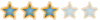# Prime Number##Prime Number

Code:
`/* Program to print prime numbers from 1 to 10*/class PrimeNumber{    public static void prime(int n)    {         int count=0;         /* This checks the number of times          * the number gets completely divided          */         for(int i=2;i<n;i++)        {              if(n%i==0)               {                  count++;              }        }        if(count==0)         {            System.out.print(n+" ");        }    }    public static void main (String[] args)     {        for(int a=1;a<=10;a++)        {            prime(a);  // Calling prime() function        }    }}`

Variable Table

 Variable Name Data Type Description count int Checks the number of times the number gets completely divided i int Used to iterate the for loop n int Formal Parameter a int Actual Parameter

______________________________________________________
It is the simplest things that are most Complicated!
Been on this forum since 3305 days!

Sh~1457Sujith
LSF ExpertPosts : 126Permissions in this forum:
You cannot reply to topics in this forum